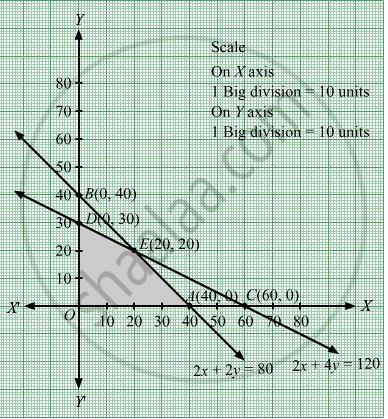# Maximize Z = 3x + 4y Subject to 2 X + 2 Y ≤ 80 2 X + 4 Y ≤ 120 - Mathematics

Maximize Z = 3x + 4y
Subject to

$2x + 2y \leq 80$
$2x + 4y \leq 120$

#### Solution

We have to maximize Z = 3x + 4y
First, we will convert the given inequations into equations, we obtain the following equations:
2x + 2y = 80, 2x + 4y = 120
Region represented by 2x + 2y ≤ 80:
The line 2x + 2y = 80 meets the coordinate axes at $A\left( 40, 0 \right)$ and  $B\left( 0, 40 \right)$ respectively. By joining these points we obtain the line 2x + 2y = 80.
Clearly (0,0) satisfies the inequation 2x + 2y ≤ 80. So,the region containing the origin represents the solution set of the inequation 2x + 2y ≤ 80.

Region represented by 2x + 4y ≤ 120:
The line 2x + 4y = 120 meets the coordinate axes at

$C\left( 60, 0 \right)$ and  $D\left( 0, 30 \right)$ respectively. By joining these points we obtain the line 2x + 4y ≤ 120.
Clearly (0,0) satisfies the inequation 2x + 4y ≤ 120. So,the region containing the origin represents the solution set of the inequation 2x + 4y ≤ 120.

The feasible region determined by the system of constraints, 2x + 2y ≤ 80, 2x + 4y ≤ 120 are as follows:The corner points of the feasible region are O(0, 0), $A\left( 40, 0 \right)$ $E\left( 20, 20 \right)$ and  $D\left( 0, 30 \right)$
The values of Z at these corner points are as follows:
 Corner point Z = 3x + 4y O(0, 0) 3 × 0 + 4 × 0 = 0 $A\left( 40, 0 \right)$ 3× 40 + 4 × 0 = 120 $E\left( 20, 20 \right)$ 3 × 20 + 4 × 20 = 140 $D\left( 0, 30 \right)$ 10 × 0 + 4 ×30 = 120

We see that the maximum value of the objective function Z is 140 which is at $E\left( 20, 20 \right)$  that means at = 20 and y = 20.
Thus, the optimal value of Z is 140.

Concept: Graphical Method of Solving Linear Programming Problems
Is there an error in this question or solution?

#### APPEARS IN

RD Sharma Class 12 Maths
Chapter 30 Linear programming
Exercise 30.2 | Q 8 | Page 32